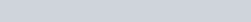ChemTalk

# Basic Math in Chemistry## Core Concepts

In this article you’ll learn the basic principles of math that are the foundation to chemistry! You’ll learn how to calculate atomic weights of atoms and their molar masses, the percent composition of an element in a compound as well how to utilize moles and Avogadro’s number! Make sure to check out the end of the article for some practice problems!

## Calculating Atomic Weight

Atomic weight is the average weight of element’s isotopes. You may also hear it referred to as atomic mass. However, atoms are extremely small, so they don’t weigh very much in our standard of weight. So, in order to make things easier we measure atomic weight in atomic mass units or amu. Most of the time, you can find the atomic weight from the periodic table. Below is a picture of an elemental symbol on the period table. The highlighted section is the atomic weight!

If you don’t have access to a periodic table but are given the weights of the different isotopes, you can still calculate the atomic weight! Take the following problem for example:

## Calculating Formula Weight

Formula weight is the overall weight of a given formula. For instance, in order to find the formula weight CO, or carbon monoxide you would add the atomic weights of carbon and oxygen. If there is a subscript, you multiply the atomic weight of that element by the subscript. For example, in CO2, or carbon dioxide, you would add the atomic weight of carbon and the atomic weight of oxygen multiplied by two. Below is an example of finding the formula weight for potassium permanganate (KMnO4) and acetic acid (C2H4O2).

Note the atomic weights are taken from the weights listed on our interactive periodic table linked earlier in the article!## Calculating Percent Composition

When calculating formula weight, you may be asked to discern what is the percent composition of a particular element in the formula. In order to do this, you take the element’s atomic weight multiplied by its atomic weight and then divide it by the overall formula weight. Below is a formula to help you remember and an example problem.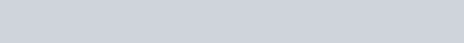We know from the previous example that the formula weight for acetic acid is 44.05 amu.

Percent Composition of H (hydrogen) in acetic acid =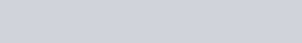In this example the Hydrogen percent composition of acetic acid is 9.144%!

## Understanding and Working with Moles in Chemistry Math

We’ve learned about amu, or the unit of measurement for atomic mass. In reality though, when we work with elements we’re working with millions of atoms! That would mean that anytime we did any calculation with atoms, we would have long and complicated numbers. In order to work around this though, scientists created another unit of measurement called moles, abbreviated as a lowercase n. A mole is definite number of things, the same way that a dozen eggs means 12 eggs.

The scientific description of a mole is the amount of a substance that has the same number of things as atoms in 12g of carbon-12. However, over the years scientists have found a much simpler term for this called Avogadro’s number. Avogadro’s number is equivalent to 6.022 x 1023. So, a mole of calcium is 6.022 x 1023 atoms, and a mole of cows is 6.022 x 1023 cows.

## Basic Chemistry Math Practice Problems

#### Problem #1

What is the formula weight of phosphoric acid, H3PO4?

#### Problem #2

What percent of sulfuric acid, H2SO4 is oxygen given that the formula weight is 98.08 amu?

#### Problem #3

Find the formula weight of nitric acid, HNO3, as well as the percent composition of nitrogen in nitric acid.

## Basic Chemistry Math Practice Problems Solutions

#1: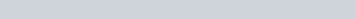#2: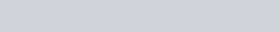#3 Formula weight: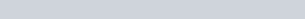Nitrogen Percent Composition: